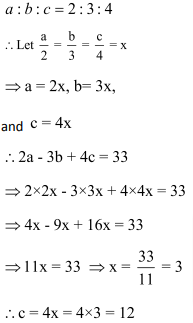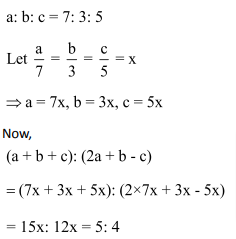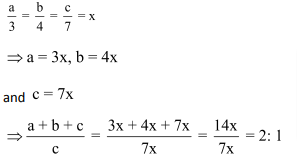We often come across the word ‘Ratio’ and are aware of its literal meaning, but how do we deal with it while solving problems? To answer that question,let us discuss Ratios point by point.

Definition:

A ratio expresses the number of times one quantity is of the other.

Mathematically speaking, if there are two quantities of the same type, i.e., A and B, then their ratio is shown as A : B. Read this as A is to B.  Here, A and B are the terms of the ratio. A is the antecedent, and B is the consequent/precedent.

For example: We assign some values to A and B,say 4 and 5.

Then, the ratio between A and B is 4 is to 5.

This can be written down as:

A: B = 4: 5 or A/B = 4/5

Basic Points for Ratio:

A ratio must be expressed between entities expressed in similar units. For example, you cannot derive a ratio between the surface area of one figure and the volume of another. This is because the units are different. For you to derive a ratio, you need to either compare the respective areas or the respective volumes of the two figures.

• You can cancel common factors to arrive at a given fraction. If there are two quantities A and B having values 3 and 6, then the ratio for the two will be 3:6 = 1:2 (after cancelling the common factor).
• If a number is multiplied to both the numerator and denominator then there will not be any change in the ratio. For example, 3:4 is the same as the 6:8, 12:16. The ratio remains the same.
• If a number, divides both the numerator and the denominator then there will not be any change in the ratio. For example, 3:4 is the same as the 3/2:4/2 = 6/2:8/2. The ratio remains the same.
• If there are two fractions and they are in a ratio then we can write them as a single fraction. For example, suppose there are two fractions like 3/4 and 5/7 and they are in ratio i.e., 3/4: 5/7. Then they can be written as 3/4 x 7/5 = 21/20.

Compound Ratio:

Ratios are compounded by multiplying together the antecedents for a new antecedent and consequents for a new consequent.

Confused? Do not be.Let us take up a practical example for the same.

Example: Find the ratio compounded for the following 3 ratios: 2:3, 5:8 and 3:7.

Solution:

The required ratio in this case is =Duplicate Ratio:

When the ratio 8: 5 is compounded with itself, then the resulting ratio, 82: 52 is called the duplicate ratio.

Triplicate Ratio:

When the ratio 8: 5 is compounded with itself twice, then the resulting ratio, 83: 53 is called the triplicate ratio.

Sub-duplicate Ratio:is the sub-duplicate ratio for 8: 5.

Sub-triplicate ratio:is the sub-triplicate ratio for 8: 5.

Inverse Ratio:

If a: b is the given ratio, then 1/a: 1/b or in other words, b:a is the inverse ratio.

To get thorough with the above concepts based on Basics of Ratio you can test yourself with the exercise given below:

Exercise:

Question 1: The ratio 21.5: 20.5 is the same as:

(1) 2: 1

(2) 3: 1

(3) 6: 1

(4) 3: 2

Solution: (1)

Concept:  ratio means a:b i.e., a/b so using it

Required ratio === 2: 1

Question 2: If x: y = 3: 2, then the ratio 2x2 + 3y2: 3x2 – 2y2 is equal to:

(1) 12: 5

(2) 6: 5

(3) 30: 19

(4) 5: 3

Solution: (3)

Use property of duplicate ratio.Divide the equation by y2.= 30: 19

Question 3: If a: b = b: c, then a4: b4 is equal to:

(1) ac: b2

(2) a2: c2

(3) c2: a2

(4) b2: ac

Solution: (2)Cross multiplicationSquare itQuestion 4: If x: y = 2: 3, then the value ofis equal to:

(1)(2)(3)(4)Solution: (2)

Given,Expression =Divide the equation by y.

Put the values [from (i)]Question 5: If x: y = 2: 1, then (x2 – y2): (x2 + y2) is

(1) 3: 5

(2) 5: 3

(3) 4: 5

(4) 5: 6

Solution: (1)

Here,Question 6: If x: y = 4: 5, then (3x + y): (5x + 3y) =

(1) 3: 5

(2) 5: 3

(3) 17: 35

(4) 35: 17

Solution: (3)

We haveQuestion 7: If m: n = 3: 2, then (4m + 5n): (4m – 5n) is equal to:

(1) 4: 9

(2) 9: 4

(3) 11: 1

(4) 9: 1

Solution: (3)So (4m + 5n): (4m – 5n) = 11: 1

Question 8: If p: q: r= 1: 2: 4, thenis equal to

(1) 5

(2) 2q

(3) 5p

(4) 4r

Solution: (3)

We have,(let)Question 9:  If a: b: c = 2: 3: 4 and 2a – 3b + 4c = 33, then the value of c is?

(1) 6

(2) 9

(3) 12

(4)Solution: (3)Question 10:  If a: b: c = 7: 3: 5, then (a + b + c): (2a + b – c) is equal to

(1) 1: 2

(2) 2: 3

(3) 3: 4

(4) 5: 4

Solution: (4)Question 11:  If a: b: c = 3: 4: 7, then the ratio (a + b + c): c is equal to:

(1) 2: 1

(2)14: 3

(3) 7: 2

(4) 1: 2

Solution: (1)

We have,### Ratio and Proportion Questions: Problems on Ratio and Proportion you should solve for competitive examination preparation

Welcome to this exercise on Problems on Ratio and Proportion. In this exercise, we build on the basic concepts for Ratio and Proportion. As you prepare for your competitive examinations, you will come across questions on Ratio and Proportion. Such questions need optimized tackling and can be solved with ease by using simple tricks and understanding the relationships highlighted in this Ratio and Proportion Questions article. The Ratio and Proportion Questions exercise comes into the picture where it gives you a chance to practice the highlighted and important concepts related to Ratio and Proportion question type.

Get Posts Like This Sent to your Email
Updates for Free Live sessions and offers are sent on mail. Don't worry: we do not send too many emails..:)
Get Posts Like This Sent to your Email
Updates for Free Live sessions and offers are sent on mail. Don't worry: we do not send too many emails..:)Get the latest updates from our side, including offers and free live updates, on email.Leverage agile frameworks to provide a robust synopsis for high level overviews.
4 Short Term Courses launched for CAT-2021. Special 10% off as launch offer.
10
days
10
hours
10
minutes
10
seconds
4 Short Term Courses launched for CAT-2021. Special 10% off as launch offer.
10
days
10
hours
10
minutes
10
seconds